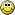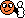# Piston Velocity and Position

#### MolaKule

Staff member
For this two-part problem we're going to use my 4.3L Vortec V6 in this example. Data: The engine's crankshaft is rotating at 1000 RPM. The Piston Stroke is 3.48 inches. To simplify the problem somewhat, neglect any connecting rod angles (for now). The usual Piston Stroke Length Ls and crank radius r, as in Ls = 2*r applies. Q1: What is the maximum velocity of any one of the pistons in Inches/Sec.? Q2: At what position in the cylinder will the piston attain its maximum velocity? This Question is not open to any Engineering Discipline or Physics Discipline.

Last edited:

Staff member

#### buck91

Wouldn't this just be [ (stroke length) * (4) * (rpm) ] / 60 seconds = inches/seconds?

#### MolaKule

Staff member
Originally Posted by buck91
Wouldn't this just be [ (stroke length) * (4) * (rpm) ] / 60 seconds = inches/seconds?
This question is similar to the chainsaw tooth velocity question: Hint: You can use one of the online calculators: Q1: What is the maximum velocity of any one of the pistons in Inches/Sec.? Q2: At what position in the cylinder will the piston attain its maximum velocity? This Question is not open to any Engineering Discipline or Physics Discipline. The centerline of the crankshaft is directly below the cylinder.

Last edited:

#### MrMoody

Assuming the centerline of the cylinder intersects the centerline of the crank, it seems fairly simple but maybe I'm missing something. If the stroke is 3.48, then so is the diameter of the big end circle. The circumference would be 3.48 x pi = ~ 10.933 inches. At 1000 rpm, that would be 10933 in/min Ã· 60 = 182.22 in/s, which would also be the maximum piston speed at 90Â° crank position. At 90Â°, the piston would be exactly halfway through its travel.

#### MrMoody

Originally Posted by MrMoody
At 90Â°, the piston would be exactly halfway through its travel.
I haven't looked at any links or google, but I don't think this is quite right, but getting it right would require knowing the rod length to do some trigonometry.

#### MolaKule

Staff member
Originally Posted by MrMoody
Originally Posted by MrMoody
At 90Â°, the piston would be exactly halfway through its travel.
I haven't looked at any links or google, but I don't think this is quite right, but getting it right would require knowing the rod length to do some trigonometry.
We're keeping it simple which is why I stated that we will neglect the crank angle for now.
Quote
To simplify the problem somewhat, neglect any connecting rod angles (for now). The usual Piston Stroke Length Ls and crank radius r, as in Ls = 2*r applies.

Last edited:

#### walterjay

The piston will be at the center point of its travel for max velocity. As for the other I cannot answer.

#### MolaKule

Staff member
Thanks to all who participated. MrMoody and Astro14 (in absentia) had the correct answers.This was another problem in the conversion of radial velocity to linear velocity as in, Vlinear = Omega*radius as two hints were given. To simplify the problem we assumed no effect of crank angle/rod/piston-pin relationships, which meant the piston is seeing only reciprocating, linear travel. Simplification also meant the connecting rod was very long so crank angles did not enter into the problem. At 0 degrees Top Dead Center and at 180 degrees Bottom Dead Center, and with the crankshaft rotating clockwise, the piston motion is virtually zero. At 90 degrees and at 270 degrees, the piston is halfway down or up the cylinder, respectively, and it is at these positions in which the piston velocity is maximum, or 182.22 inches per second. For the next QOTD, we will include crank angle.